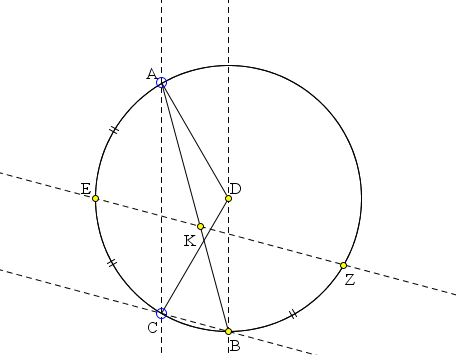# Circle Division by Euclid and Leonardo

Euclid of Alexandria, the famous author of the Elements, is reputed to have authored at least 8 more books. Some, like the Elements, have been preserved almost in entirety, some have been lost, one - On Divisions (of figures) - reached the New Age through a partial Arabic translation. In the early 1900s, R. C. Archibald, Professor at Brown University, undertook a restoration of the text based on that translation and Leonardo Fibonacci's Practica Geometriae. Archibald's book is now available online at The Cornell Library Historical Mathematics Monographs. (I thank Douglas Rogers for pointing me to the wonderful resource.)

The present page is devoted to the

### Proposition 29

To draw in a given circle two parallel lines cutting off a certain fraction from the circle.

The proposition comes with a comment that in Leonardo's book the fraction is taken to be 1/3 and the wording of the solution in the translation is "not wholly satisfactory." Here how it goes:

Let the certain fraction be one-third, and the circle be ABC. It is required to do that which is about to be explained.Construct the side of the triangle (regular) inscribed in the circle. Let this be AC. Draw the two lines AD, DC and draw through the point D (center of the circle) a line parallel to the line AC, such as DB. Draw the line CB. Divide the arc AC into two equal parts at the point E, and draw from the point E the chord EZ parallel to the line BC. Finally draw the line AB. I say that we have two parallel lines EZ, CB cutting off a third of the circle ABC, viz., the figure ZBCE.

Demonstration. The line AC being parallel to the line DB, the triangle DAC will be equal to the triangle BAC; add to each the segment of the circle AEC; the whole figure DAEC will be equal to the whole figure BAEC. But the figure BAEC is one-third of the circle. Consequently the figure DAEC is also one-third of the circle. Since EZ is parallel to CB, the arc EC will be equal to the arc BZ; but EC is equal to EA, hence EA equals ZB. Add to this equal parts the arc ECB; the whole arc AB will be equal to the common segment of the circle ECBZ. Taking away the common segment BC, there remains the figure EZBC equal to the figure BAEC. But the figure BAEC was one-third of the circle ABC. Then the figure EZBC is one-third of the circle ABC; which was to be demonstrated.

When it is required to cut off a quarter of a circle, or a fifth or any other definite fraction, by means of two parallel lines, we construct in this circle the side of a square or of the pentagon (regular) inscribed in the circle and we draw from the centre to the extremities of this side the two straight lines as above. (The remainder of) the construction will be analogous to that which has gone before.

It must be added that the construction of regular polygons is not always possible. E.g., the heptagon is not constructible with ruler and compass.

### References

1. R. C. Archibald, Euclid's Book On Divisions of Figures, Cambridge University Press, 1915